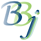# BBjNumber

## Description

In BBj 7.0 and higher, the BBjNumber object defines an object-oriented interface to traditional BBx numbers. It is not a java.lang.Number, but it implements the conversion methods defined by that class. This API is primarily designed to facilitate interaction with Java libraries.

## Creation

BBjAPI > BBjNumber

All numeric values created in BBj are BBjNumbers. To access BBjNumber methods, copy a BBj numeric value to an object variable:

number! = num("1")
print number!.booleanValue()

## Methods of BBjNumber

Return Value

Method

boolean

byte

double

boolean

equals(Object val)

float

int

hashCode()

int

intValue()

long

short

string

toString()

## Remarks

BBjInts are BBjNumbers that throw an error when a non-integer value is assigned.

None.

## Example

 rem ' BBjNumber print "BBjNumber sample code" n! = 3.14159 print "n!=",n!.toString() print "n!.booleanValue()=",n!.booleanValue() print "n!.byteValue()=",n!.byteValue() print "n!.doubleValue()=",n!.doubleValue() print "n!.equals(3.14159))=",n!.equals(3.14159) print "n!.floatValue()=",n!.floatValue() print "n!.hashCode()=",n!.hashCode() print "n!.intValue()=",n!.intValue() print "n!.longValue()=",n!.longValue() print "n!.shortValue()=",n!.shortValue()